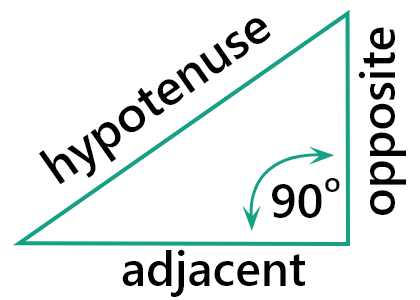# Hypotenuse Calculator

To find the hypotenuse of a triangle, enter the Adjacent side (a), Opposite side (b), and click calculate button using hypotenuse calculator

Formula:
c = a2 + b2

Give Us Feedback

## Hypotenuse Calculator

Hypotenuse calculator is used to finding the length of the hypotenuse of a right-angle triangle by taking the two sides of the triangle. The sides are adjacent and opposite denoted by a and b respectively.

## What is the hypotenuse?

The hypotenuse is the longest side of a right-angle triangle that is opposite to the right angle (perpendicular line). The term hypotenuse is a part of the Pythagorean theorem. It is easily calculated by using the Pythagorean theorem## Formula of hypotenuse

The hypotenuse is the square root of the square of the adjacent side plus the square of the opposite side. It is denoted by c. The general equation is:

Pythagorean theorem: c2 = a2 + b2

Hypotenuse = c = √(a2 + b2)

## How to calculate hypotenuse?

The calculation of the hypotenuse can be done easily by using the above hypotenuse of a triangle calculator. For solving manually follow the below example.

Example

Find the hypotenuse of a right triangle if the adjacent (a) is 12cm and the opposite (b) is 5cm.

Solution

Step 1: Write the given information.

Opposite (b) = 5cm

Hypotenuse (c) =?

Step 2: Take the general formula for finding the hypotenuse and substitute the given values.

Hypotenuse = c = √(a2 + b2)
= √(122 + 52)
= √(144 + 25)
= √(169)
= 13cm

### Math Tools